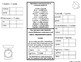# Converting Units of MeasurementSubject
Resource Type
File Type
PDF (1 MB|10 pages)
Standards
\$2.00
Also included in:
1. This product includes all of my Unit 2: Ratios and Proportional Relationships foldables and practice. Includes:Unit Standards and Goal SheetConverting Fractions, Decimals and PercentsRatios, Rates and Equivalent RatesThe Coordinate PlaneGraphing Equivalent Ratios and RatesSolving Problems with Unit
\$17.00
\$11.90
Save \$5.10
• Product Description
• Standards
This resource is perfect for your interactive notebooks. Provides a review of customary and metric units and common approximate conversions between the two systems. Students complete 6 conversion practice problems using ratio table after completing an example problem with teacher. Additional practice page and answer key provided as well.

This foldable is easy and requires no cutting, how cool!. Simply print pages 3 and 4 two-sided, flipped on the short edge. Take the bottom right corner and fold up to the left edge. Line up the edge of the paper under the corner of the table on the inside. Then take the top right edge and fold over to line up along the left edge. All set up! Answer key is provided on page 5. Don’t forget to earn your TPT credits by rating my product. Email me with any questions at baileysmathnotebooks@gmail.com.

Bundle and Save

Unit 1: Fraction and Decimal Operations

Unit 2: Ratios and Proportional Relationships

Unit 3: Properties and Expressions

Unit 4: Equations and Inequalities

Unit 5: Rational Numbers

Unit 6: Geometry

Unit 7: Statistics

Also Check out my 6th Grade Math Complete Interactive Notebook Bundle
Use ratio reasoning to convert measurement units; manipulate and transform units appropriately when multiplying or dividing quantities.
Use ratio and rate reasoning to solve real-world and mathematical problems, e.g., by reasoning about tables of equivalent ratios, tape diagrams, double number line diagrams, or equations.
Total Pages
10 pages